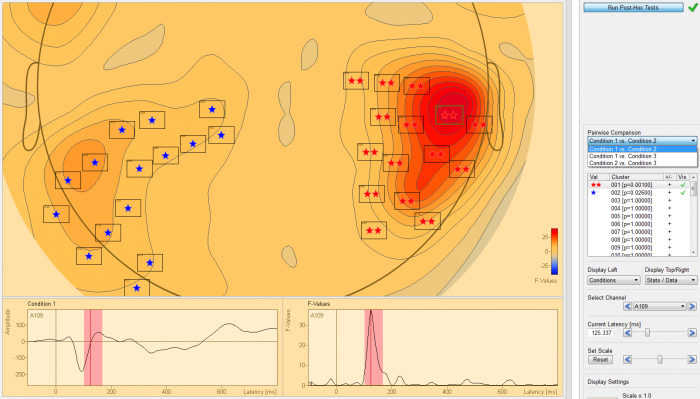# Post-hoc Testing

## Post-hoc Testing

It is necessary to run a post-hoc test when a main effect in a one-way ANOVA / ANCOVA with more than two groups / conditions becomes significant. BESA Statistics uses Scheffe’s test for this purpose. Scheffe’s test computes F-values for all pairwise comparisons of groups / conditions.

As each permutation is associated with different clusters, and in consequence different cluster F-values, Scheffe’s test must also be computed for each permutation. A new distribution of Scheffe’s test F-values is built and p-values are derived directly from the computed distribution (see Permutation Testing).

As Scheffe’s test runs pairwise comparisons, the permutation test based on Scheffe’s F-values is repeated several times. As an example, if 3 groups / conditions are compared, 3 permutation tests need to be computed. In the case of 4 groups / conditions, 6 permutation tests must be run. In order to correct for this multiple comparison problem, BESA Statistics combines the Bonferroni Holm method with the permutation Scheffe’s test.

### Post-hoc testing using Scheffe’s test#### BESA Statistics

• Private: Features